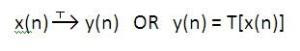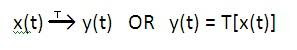# Properties of a system

## Properties of a system

### System:

A system is a physical device (or an algorithm) which performs required operation on a discrete time signal.

A discrete time signal is represented as shown in figure below.

• Here x(n) input discrete time signal applied to the system. It is also called as excitation.

• The system operates on discrete time signal. This is called as procession of input signal, x(n).

• Output of the system is denoted by y(n). It is also called as response of the system.

### Notation:

When input signal is passed through the system then it is represented by following notation:Here T is called as transformation operation. Similarly for continuous time system:### Example:

A filter is good example of a system. A signal containing noise is applied to the input of the filter. This is an input signal to the system. The filter cancels or attenuates noise signal. This is the processing of the signal. A noise-free signal obtained at the output of the filter is called as response of the system.

### Classification (or properties) of the system:

Properties of systems are with respect to input and output signal. For the simplicity we will assume that the system has single input and single output. But the same explanation is valid for system having multiple inputs and outputs. Important properties (or classification) of the systems are listed below:

Now based on the properties, the systems can be classified as follows:

We will see these different types (i.e. properties) of a system in upcoming posts. So stay connected.

### You may also like:

1.